# Diagonal Matrix

## Diagonal Matrix in C and C++ Language with Examples:

In this article, I am going to discuss Diagonal Matrix in C and C++ Language with Examples. Please read our previous article, where we give a brief Introduction to Matrices.

##### Diagonal Matrix:

The following is a diagonal matrix. We have taken a diagonal matrix of order 5×5. That is 5 rows and 5 columns. Here we can see that most of the numbers are ‘0’ and only the elements in the diagonal are non-zero. The important thing is other than diagonal all elements must be ‘0’. Then only we say it is a diagonal matrix.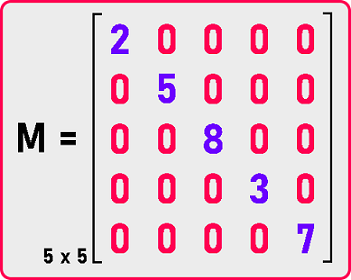If we have non-zero elements other than diagonal then that will not be a diagonal matrix. Below is not a diagonal matrix.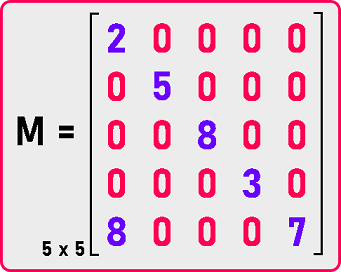The important condition is all the elements other than diagonal must be ‘0’. Let us see how we can define this. For Diagonal Matrix, the condition is:If row number and column number are the same then the value will be non-zero and if row number and column number are different then the value will be ‘0’ in the diagonal matrix.

Now if we have to represent a diagonal matrix in a program then for a matrix, we have to take a two-dimensional array. But if we take a two-dimensional array of size 5×5, then most of the elements will be zeros.If we take a two-dimensional array for storing this matrix then most of the elements are zeros and if these are integers an integer is taking 2 bytes, then total how many bytes of memory this array is consuming. There are 5×5 elements that is 25 elements are there and each element is taking 2 bytes. Then it will take 50 bytes of memory.

If we are storing this matrix in 50 bytes of memory then storage of ‘0’ elements is unnecessary. It is wasting space as well as when we’ll processing on a diagonal matrix-like if we are adding 2 diagonal matrices then adding ‘0’ is of no use or if we are multiplying 2 diagonal matrices then multiplication with zeros is of no use. So, we will be wasting time in the processing of ‘0’. So, the idea here is that we want to store only non-zero elements so how we can store only non-zero elements?

For storing non-zero elements we can take just a single dimension array and store these elements. Now let us see how we can represent a diagonal matrix and adjust it in a single dimension array.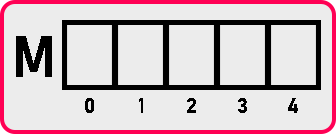We would take a single dimension array size 5 because we have only 5 non-zero elements This study starting index is 0 but here if you observe I have taken then this is from 1 onwards. We will take all the indices starting from 0 onwards to represent a matrix. So let us store the non-zero elements in the array as:Here we have stored only non-zero elements. Now let us see how we can access these elements from a single dimension array if we want to access them

1. If we want to access M [0, 0], this element is present on the 0th index in the array.
2. If we want to access M [1, 1], this element is present on the 1 index in the array.

We have stored only non-zero elements in the array. If (i == j) in the matrix, then we can get that element from the array which will index at M [i] or M [j]. Here, ‘i’ represents the number of rows in the matrix and ‘j’ represents the number of columns in the matrix. Now we will see how we can write a C or C++ program code for representing the diagonal matrix. Let see the code part.

##### Diagonal Matrix Code in C Language:
```#include <stdio.h>
struct Matrix
{
int B;
int n;
};

void Set (struct Matrix *m, int i, int j, int y)
{
if (i == j)
m->B[i - 1] = y;
}

int Get (struct Matrix m, int i, int j)
{
if (i == j)
return m.B[i - 1];
else
return 0;
}

void Display (struct Matrix m)
{
int i, j;
printf ("Matrix is: \n");

for (i = 0; i < m.n; i++)
{
for (j = 0; j < m.n; j++)
{
if (i == j)
printf ("%d ", m.B[i]);
else
printf ("0 ");
}
printf ("\n");
}
}

int main ()
{
struct Matrix M;
M.n = 5;

Set (&M, 1, 1, 2);
Set (&M, 2, 2, 5);
Set (&M, 3, 3, 8);
Set (&M, 4, 4, 3);
Set (&M, 5, 5, 7);

Display (M);
return 0;
}
```
###### Output: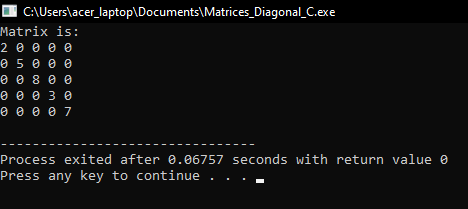##### Diagonal Matrix Code in C++ Language:
```#include <iostream>
using namespace std;
class DiagonalMatrix
{
private:
int *B;
int n;

public:
DiagonalMatrix ()
{
n = 2;
B = new int;
}

DiagonalMatrix (int n)
{
this->n = n;
B = new int[n];
}

~DiagonalMatrix ()
{
delete[]B;
}

int GetDimension ()
{
return n;
}

void Set (int i, int j, int y);
int Get (int i, int j);
void Display ();
};

void DiagonalMatrix::Set (int i, int j, int y)
{
if (i == j)
B[i - 1] = y;
}

int DiagonalMatrix::Get (int i, int j)
{
if (i == j)
return B[i - 1];
return 0;
}

void DiagonalMatrix::Display ()
{
cout << "\nMatrix is: " << endl;
for (int i = 1; i <= n; i++)
{
for (int j = 1; j <= n; j++)
{
if (i == j)
cout << B[i - 1] << " ";
else
cout << "0 ";
}
cout << endl;
}
}

int main ()
{
int d;
cout << "Enter Dimensions: ";
cin >> d;

DiagonalMatrix dm (d);

int x;
cout << "Enter All Elements: " << endl;
for (int i = 1; i <= d; i++)
{
for (int j = 1; j <= d; j++)
{
cin >> x;
dm.Set (i, j, x);
}
}

dm.Display ();
return 0;
}
```
###### Output: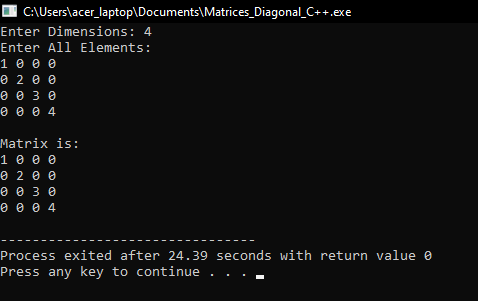In the next article, I am going to discuss Lower Triangular Matrix Row-Major Mapping in C and C++ Language with Examples. Here, in this article, I try to explain Diagonal Matrix in C and C++ Language with Examples and I hope you enjoy this Diagonal Matrix in C and C++ Language with Examples article.